# BACKGROUND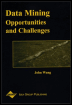Chapter II - Control of Inductive Bias in Supervised Learning Using Evolutionary Computation A Wrapper-Based Approach Data Mining: Opportunities and Challenges by John Wang (ed) Idea Group Publishing 2003Brought to you by Team-Fly

### Key Problem: Automated Control of Inductive Bias

We first focus on development of a new learning system for spatiotemporal KD. The KD performance element in this problem is not just analytical but includes decision support through model visualization and anomaly detection.

The problems we face are threefold and are surveyed in the above sections on current and related work. First, we must address relevance determination to determine what sensor data channels are useful for a particular KD objective and data set. This problem is related to the so-called curse of dimensionality wherein an overabundance of input variables makes it difficult to learn the prediction, classification, or pattern recognition element. Second, the task of identifying hyperparameters of the KD system is subject to deceptivity and instability because bias optimization in general introduces a new level of combinatorial complexity to the problem of inductive learning. Third, the very large spatiotemporal databases we are dealing with are highly heterogeneous, arising from many disparate sources such as global positioning systems (GPS), surveying, sensors such as radar, and historical databases; this heterogeneity presents the advantage of type information that can help constrain dimensionality but also aggravates the problem of relevance determination because including irrelevant sensors can lead to severe inefficiencies in data acquisition, interpretation of visual results, and the learning component.

In Hsu, Ray, and Wilkins et al.(2000), we address these problems through a framework called composite learning that is depicted in Figure 1. We define a composite learning system to be a committee machine (Haykin, 1999) designed to improve the performance of a collection of supervised inductive learning algorithms with specific parameters for representation and preference bias (Mitchell, 1997), over multivariate possibly heterogeneous data. The open research problem I discuss is how composite learning systems can be applied to automatically improve representations of complex learning problems.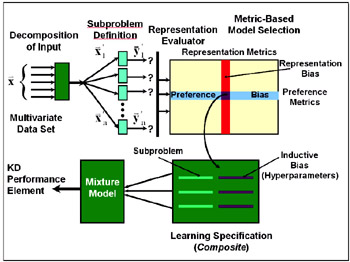Figure 1: A composite learning framework.

Composite learning provides a search-based and validation-based procedure for controlling the inductive bias, specifically the total problem specification, of systems for learning from decomposable, multi-attribute data sets. The central elements of this system are: decomposition of input, metric-based model selection, and a mixture model for integration of multiple submodels. In recent research, I applied composite learning to audio signal classification (Ray & Hsu, 1998) and crop condition prediction, the central component of a monitoring problem (Hsu, 1998; Hsu et al., 2000). Given a specification for decomposed i.e., selected or partitioned subsets of input variables, new intermediate concepts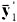can be formed by unsupervised learning. For this step we have used Gaussian radial-basis functions or RBFs (Ray & Hsu, 1998) and self-organizing maps (Kohonen, 1990). The newly defined problem or problems can then be mapped to one or more appropriate hypothesis languages (model specifications). We have developed a high-level algorithm for tuning explicit parameters that control representation and preference bias, to generate this specification of a composite. This algorithm is used by Hsu et al., (2000) to select components for a hierarchical mixture network (specialist-moderator network) and train them for multi-strategy learning. A data fusion step occurs after individual training of each model. The system incorporates attribute partitioning into constructive induction to obtain multiple problem definitions (decomposition of learning tasks); applies metric-based model selection over subtasks to search for efficient hypothesis preferences; and integrates these techniques in a data fusion (mixture estimation) framework.

The metrics we have derived for controlling preference bias in hierarchical mixture models are positively correlated with learning performance by a particular learning method (for a learning problem defined on a particular partitioning of a time series). This makes them approximate indicators of the suitability of the corresponding mixture model and the assumption that the learning problem adheres to its characteristics (with respect to interaction among subproblems). Thus, preference bias metrics may be used for partial model selection.

Although this approach has yielded positive results from applying composite learning to KD, the breadth of domains for which this framework has been tested is still limited. A STETchallenge and opportunity is the application of composite learning to learning problems in precision agriculture, specifically the illustrative example in and the problem of soil fertility mapping, which generates a map of quantitative fertility estimates from remotely sensed, hydrological, meteorological, wind erosion, and pedological data. One purpose of generating such maps is to control variable-rate fertilizer application to increase yield with minimal environmental impact. Test bed for heterogeneous data mining abound in the literature and are becoming freely available.

In past and current research, we have achieved empirical improvement in constructive induction in several ways in which we propose to further generalize and systematically validate. First, we found that decomposition of learning tasks using techniques such as attribute partitioning or ensemble selection can help reduce variance when computational resources are limited. We conjecture that this may be useful in domains such as real-time intelligent systems, where deadlines are imposed on training and inference time.

Current techniques such as automated relevance determination, feature selection, and clustering tend to address the problem of constructive induction in isolated stages rather than as an integrative mechanism for transforming the data input and output schemata into a more tractable and efficient form. As outlined in the previous section, we address this by combining search-based combinatorial optimization, statistical validation, and hierarchical abstraction into the coherent framework of composite learning.

Furthermore, many complex KDD problems can be decomposed on the basis of spatial, temporal, logical, and functional organization in their performance element. Techniques such as model selection and ensemble learning have been used to systematically identify and break down these problems, and, given a specification of a modular learning system, hierarchical mixture estimation techniques have been used to build pattern recognition models by parts and integrate them. The challenge is how to isolate prediction or classification models. The author has identified several low-order Box-Jenkins (autoregressive integrated moving average, also known as ARMA or ARIMA) process models (Box et al., 1994) that can be isolated from heterogeneous historical data, based on quantitative metrics (Hsu, 1998). Composite learning can be applied to derive a complete committee machine specification from data to learn intermediate predictors (e.g., temporal artificial neural networks such as simple recurrent networks and time-delay neural networks). We believe that this approach can discover other hierarchical organization such as embedded clusters (Hsu et al., 2002), factorial structure (Ray & Hsu, 1998), and useful behavioral structure, which we shall outline in the next section on evolutionary computation for KD. The proposed research is therefore not specific to time series.

The line of research that we have described in this section shall lead to the development of techniques for making inductive learning more robust by controlling inductive bias to increase generalization accuracy. We propose to use my framework, composite learning, for specifying high-level characteristics of the problem representation to be tuned in a systematic way. The next section presents a specific combinatorial optimization technique for tuning these hyperparameters using validation and other criteria.

### Evolutionary Computation Wrappers for Enhanced KD

Over the past three years, we have been engaged in the development of a novel system for combinatorial optimization in KD from complex domains, which uses evolutionary computation genetic algorithms (GA) and genetic programming (GP) to enhance the machine learning process. Mechanisms for KD enhancement that use the empirical performance of a learning function as feedback are referred to in the intelligent systems literature as wrappers (Kohavi & John, 1997). Our objective at this stage of the research is to relax assumptions we have previously made regarding two aspects of automatically improving the representation of learning problems. First, we seek to generalize the structure of the mapping between the original and improved representations, not restricting it merely to feature selection or construction. Second, we seek to design a framework for automatic discovery of hierarchical structure in learning problems, both from data and from reinforcements in problem solving environments. The key contribution of this component of the research is to make the automatic search for representations more systematic and reproducible by putting it into an engineering framework.

### Problems: Attribute Subset Selection and Partitioning

This section introduces the attribute partitioning problem and a method for subproblem definition in multi-attribute inductive learning.

### Attribute-Driven Problem Decomposition for Composite Learning

Many techniques have been studied for decomposing learning tasks to obtain more tractable subproblems and to apply multiple models for reduced variance. This section examines attribute-based approaches for problem reformulation, which start with restriction of the set of input attributes on which the supervised learning algorithms will focus. First, this chapter presents a new approach to problem decomposition that is based on finding a good partitioning of input attributes. Previous research on attribute subset selection (Kohavi & John, 1997), is highly relevant, though directed toward a different goal for problem reformulation; this section outlines differences between subset selection and partitioning and how partitioning may be applied to task decomposition. Second, this chapter compares top-down, bottom-up, and hybrid approaches for attribute partitioning, and considers the role of partitioning in feature extraction from heterogeneous time series. Third, it discusses how grouping of input attributes leads naturally to the problem of forming intermediate concepts in problem decomposition. This mechanism defines different subproblems for which appropriate models must be selected; the trained models can then be combined using classifier fusion models adapted from bagging (Breiman, 1996), boosting (Freund & Schapire, 1996), stacking (Wolpert, 1992), and hierarchical mixture models (Jordan & Jacobs, 1994).

### Overview of Attribute-Driven Decomposition

Figure 2 depicts two alternative systems for attribute-driven reformulation of learning tasks (Benjamin, 1990; Donoho, 1996). The left-hand side, shown with dotted lines, is based on the traditional method of attribute subset selection (Kohavi & John, 1997). The right-hand side, shown with solid lines, is based on attribute partitioning, which is adapted in this chapter to decomposition of time series learning tasks. Given a specification for reformulated (reduced or partitioned) input, new intermediate concepts can be formed by unsupervised learning (e.g., conceptual clustering); the newly defined problem or problems can then be mapped to one or more appropriate hypothesis languages (model specifications). The new models are selected for a reduced problem or for multiple subproblems obtained by partitioning of attributes; in the latter case, a data fusion step occurs after individual training of each model.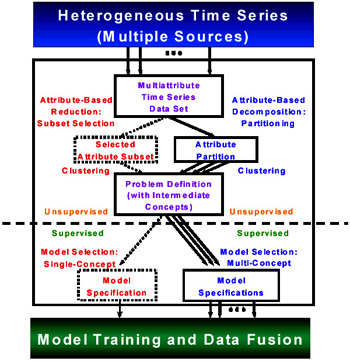Figure 2: Systems for attribute-driven unsupervised learning and model selection.

### Subset Selection and Partitioning

Attribute subset selection, also called feature subset selection, is the task of focusing a learning algorithm's attention on some subset of the given input attributes, while ignoring the rest (Kohavi & John, 1997). In this research, subset selection is adapted to the systematic decomposition of learning problems over heterogeneous time series. Instead of focusing a single algorithm on a single subset, the set of all input attributes is partitioned, and a specialized algorithm is focused on each subset. While subset selection is designed for refinement of attribute sets for single-model learning, attribute partitioning is designed specifically for multiple-model learning. This new approach adopts the role of feature construction in constructive induction (Michalski, 1983; Donoho, 1996), as depicted in Figure 2. It uses subset partitioning to decompose a learning task into parts that are individually useful, rather than to reduce attributes to a single useful group. This permits multiple-model methods such as bagging (Breiman, 1996), boosting (Freund & Schapire, 1996), and hierarchical mixture models (Jordan & Jacobs, 1994) to be adapted to multi-strategy learning.

### Partition Search

For clarity, I review the basic combinatorial problem of attribute partitioning. First, consider that the state space for attribute subset selection grows exponentially in the number of attributes n: its size is simply 2n. The size of the state space for n attributes is Bn, the nth Bell number, defined as follows: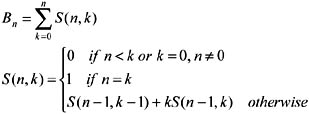Thus, it is impractical to search the space exhaustively, even for moderate values of n. The function Bn is ω(2n) and o(n!), i.e., its asymptotic growth is strictly faster than that of 2n and strictly slower than that of n!. It thus results in a highly intractable evaluation problem if all partitions are considered. Instead, a heuristic evaluation function is used so that informed search methods (Russell & Norvig, 1995) may be applied. This evaluation function is identical to the one used to prescribe the multi-strategy hierarchical mixture of experts (MS-HME) model; therefore, its definition is deferred until the next section.

The state space for of a set of five attributes consists of 52 possible partitions. We shall examine a simple synthetic problem learning problem, modular parity, that can be used to test search algorithms for the optimum partition. As the parity problem, a generalization of XOR to many variables, demonstrates the expressiveness of a representation for models or hypotheses in inductive learning (and was thus used to illustrate the limitations of the perceptron), the modular parity problem tests the expressiveness and flexibility of a learning system when dealing with heterogeneous data.

### Subproblem Definition

This section summarizes the role of attribute partitioning in defining intermediate concepts and subtasks of decomposable time series learning tasks, which can be mapped to the appropriate submodels.

### Intermediate Concepts and Attribute-Driven Decomposition

In both attribute subset selection and partitioning, attributes are grouped into subsets that are relevant to a particular task: the overall learning task or a subtask. Each subtask for a partitioned attribute set has its own inputs (the attribute subset) and its own intermediate concept. This intermediate concept can be discovered using unsupervised learning algorithms, such as k-means clustering. Other methods, such as competitive clustering or vector quantization (using radial basis functions (Lowe, 1995; Hassoun, 1995; Haykin, 1999), neural trees (Li et al., 1993), and similar models (Duda et al., 2000; Ray & Hsu, 1998), principal components analysis (Watanabe, 1985; Hassoun, 1995; Haykin, 1999), Karhunen-Lo ve transforms (Watanabe, 1985, Hassoun, 1995), or factor analysis (Watanabe, 1985; Duda et al., 2000), can also be used.

Attribute partitioning is used to control the formation of intermediate concepts in this system. Attribute subset selection yields a single, reformulated learning problem (whose intermediate concept is neither necessarily different from the original concept, nor intended to differ). By contrast, attribute partitioning yields multiple learning subproblems (whose intermediate concepts may or may not differ, but are simpler by design when they do differ).

The goal of this approach is to find a natural and principled way to specify how intermediate concepts should be simpler than the overall concept. In the next section, two mixture models are presented: the Hierarchical Mixture of Experts (HME) of Jordan and Jacobs (1994), and the Specialist-Moderator (SM) network of Ray and Hsu (Ray & Hsu, 1998; Hsu et al., 2000). The following sections explain and illustrate why this design choice is a critically important consideration in how a hierarchical learning model is built, and how it affects the performance of multi-strategy approaches to learning from heterogeneous time series. The mechanisms by which HME and SM networks perform data fusion, and how this process is affected by attribute partitioning, are examined in both theoretical and experimental terms in this chapter. Finally, a survey of experiments by the author investigates the empirical effects of attribute partitioning on learning performance, including its indirect effects through intermediate concept formation.

### Role of Attribute Partitioning in Model Selection

Model selection, the process of choosing a hypothesis class that has the appropriate complexity for the given training data (Geman et al., 1992; Schuurmans, 1997), is a consequent of attribute-driven problem decomposition. It is also one of the original directives for performing decomposition (i.e., to apply the appropriate learning algorithm to each homogeneous subtask). Attribute partitioning is a determinant of subtasks, because it specifies new (restricted) views of the input and new target outputs for each model. Thus, it also determines, indirectly, what models are called for. This system organization may be described as a wrapper system cf. (Kohavi & John, 1997) whose primary adjustable parameter is the attribute partition. A second parameter is a high-level model descriptor (the architecture and type of hierarchical classifier fusion model).

### Machine Learning Methodologies: Models and Algorithms

#### Recurrent Neural Networks and Statistical Time Series Models

SRNs, TDNNs, and gamma networks (Mehrotra et al., 1997) are all temporal varieties of artificial neural networks (ANNs). A temporal na ve Bayesian network is a restricted type of Bayesian network called a global knowledge map as defined by Heckerman (1991), which has two stipulations. The first is that some random variables may be temporal (e.g., they may denote the durations or rates of change of original variables). The second is that the topological structure of the Bayesian network is learned by na ve Bayes. A hidden Markov model (HMM) is a stochastic state transition diagram whose transitions are also annotated with probability distributions over output symbols (Lee, 1989).

The primary criterion used to characterize a stochastic process in my multi-strategy time series learning system is its memory form. To determine the memory form for temporal ANNs, two properties of statistical time series models are exploited. The first property is that the temporal pattern represented by a memory form can be described as a convolutional code. That is, past values of a time series are stored by a particular type of recurrent ANN, which transforms the original data into its internal representation. This transformation can be formally defined in terms of a kernel function that is convolved over the time series. This convolutional or functional definition is important because it yields a general mathematical characterization for individually weighted "windows" of past values (time delay or resolution) and nonlinear memories that "fade" smoothly (attenuated decay, or depth) (Mozer, 1994; Princip & Lefebvre, 2001; Princip & deVries, 1992). It is also important to metric-based model selection, because it concretely describes the transformed time series that we should evaluate in order to compare memory forms and choose the most effective one. The second property is that a transformed time series can be evaluated by measuring the change in conditional entropy (Cover & Thomas, 1991) for the stochastic process of which the training data is a sample. The entropy of the next value conditioned on past values of the original data should, in general, be higher than that of the next value conditioned on past values of the transformed data. This indicates that the memory form yields an improvement in predictive capability, which is ideally proportional to the expected performance of the model being evaluated.

Given an input sequence x(t) with components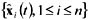, its convolution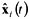with a kernel function ci(t) (specific to the ith component of the model) is defined as follows: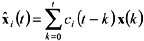(Each x or xi value contains all the attributes in one subset of a partition.)

Kernel functions for simple recurrent networks, Gamma memories, and are presented in the context of convolutional codes and time series learning by Mozer (1994), Mehrotra et al. (1997), and Hsu (1998). The interested reader may also refer to data sets such as the Santa Fe corpus (Gershenfeld & Weigend, 1994) and ANN simulation software for additional information; readers new to this family of learning models are encouraged to experiment with such test corpora and codes in order to gain basic experience.

### Evolutionary Computation: Genetic Algorithms and Genetic Programming

The notion of using evolutionary computation to improve the representation of learning problems in KD draws from foundational work on controlling genetic algorithms and finds applications in evolutionary control and data mining using genetic algorithms as inducers.

In the field of evolutionary computation, many aspects of the genetic coding and evolutionary system can be tuned automatically. Much of the recent research has focused on this meta-optimization problem and has led to both theoretical and empirical results on population sizing (Horn, 1997), probability of selection, crossover, and mutation (Goldberg, 1998), and parallel, distributed load balancing in genetic algorithms (Cantu-Paz, 1999). Genetic algorithms that tune some of their own hyperparameters are referred to in the literature as parameterless (Harik & Lobo, 1997). This idea has also been used to develop genetic wrappers for performance enhancement in KD, an innovation dating back to the first applications of genetic algorithms to inductive learning (Booker, Goldberg, & Holland, 1989; Dejong et al., 1993; Goldberg, 1989).

We seek to optimize the representation and preference biases of a learning system for KD. Therefore, we are interested in four kinds of hyperparameter: input descriptors, output descriptors, specifications for what kind of committee machine or ensemble architecture to use, and control variables for the search algorithm (the choice of search algorithm itself, heuristic coefficients, and hyperparameters in various learning frame-works). The first three kinds of hyperparameter control are representation bias, the fourth, preference bias. (Witten & Frank, 2000) This distinction is important in our study of evolutionary computation because it generates requirements for coding and fitness evaluation in our specification of combinatorial optimization problems. For example, finding intermediate learning targets can be formulated as an unsupervised learning problem, and the gene expression of an evolved selector, partition, or construction rule or program for describing these target outputs shall differ from that for inputs.

Koza (1992) defines five specification components for a GP system: determining the terminal set, function set, fitness cases or evaluation function, termination conditions, and result. The process of determining these drives the design of a GP-based wrapper. In data mining with evolutionary algorithms, many direct approaches have been made toward constructive induction; selecting and extracting features is very natural with a genetic algorithm because the hyperparameters (e.g., feature subsets) can be encoded as bit strings and, provided the proper parallel and distributed computing system is used, the task of evaluating fitness based upon model criteria and statistical validation data is trivially parallelizable. Similarly, with the proper encoding of synthetic variables as symbolic (e.g., logical or arithmetic) expressions over the original ground variables, GP is well suited to performing feature construction by combinatorial optimization.

There is a extensive but diffuse literature on hierarchical learning (especially in areas of biologically inspired computing where it is studied in contexts of neural modularity and hierarchy; niching, speciation, and demes) and artificial societies. In contrast, the concept of divide-and-conquer algorithms is pervasively and thoroughly studied. This line of research aims toward raising the understanding of layered learning in soft computing to such a level, particularly for evolutionary computation in KD and reinforcement learning over large spatial and temporal databases.

S is a recurrence known as the Stirling Triangle of the Second Kind. It counts the number of partitions of an n-set into k classes (Bogart, 1990).

 Brought to you by Team-Fly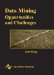Data Mining: Opportunities and Challenges
ISBN: 1591400511
EAN: 2147483647
Year: 2003
Pages: 194
Authors: John Wang

flylib.com © 2008-2017.
If you may any questions please contact us: flylib@qtcs.net